## research papersBIOLOGICALCRYSTALLOGRAPHY
ISSN: 1399-0047
Volume 56| Part 10| October 2000| Pages 1324-1331

## Low-resolution phase extension using wavelet analysis

aDepartment of Physics, York University, Heslington, York, YO10 5DD, England, and bDepartment of Chemistry, York University, Heslington, York, YO10 5DD, England
*Correspondence e-mail: julie@ysbl.york.ac.uk

(Received 25 April 2000; accepted 27 July 2000)

A method to extend low-resolution phases is presented which uses histogram matching not only of the electron density, but also of histograms obtained from the different levels of detail provided by the wavelet transform of the electron density. Statistical values for the wavelet coefficients can be predicted and depend only on the resolution and solvent content. Therefore, new details can be added to an electron-density map by matching the values of the wavelet coefficients to those predicted for an increased resolution. The positions of the new details are also guided by the diffraction pattern. In this way, the resolution can be increased gradually; on a number of trial structures of different size, solvent percentage and space group, it has been possible to extend the phasing from 10 Å to around 6–7 Å.

### 1. Introduction

An electron-density map which can be interpreted in terms of an atomic model requires both the phases and amplitudes of the structure factors. However, only the diffraction amplitudes can be measured experimentally, giving rise to the so-called phase problem' in crystallography. The standard methods of multiple isomorphous replacement (MIR) and multiple-wavelength anomalous dispersion (MAD) can provide the required phases, although isomorphous derivatives or anomalous scatterers are not always easily obtained. Similarly, there is often no suitable starting model for structure solution by molecular replacement and a phasing scheme is required which does not depend upon any special circumstances or conditions. A method for the complete ab initio determination of macromolecular crystal structures using X-ray diffraction data from a single crystal may be divided into the following three stages.

 (i) Determine an initial image of the molecule at about 10–­15 Å resolution to show the molecular envelope. (ii) Increase the resolution of this image to about 4 or 5 Å to resolve the tertiary structure. (iii) Use image-processing techniques to improve the ­electron-density map and increase the resolution to that of the X-­ray data.
The final stage is now well developed. Image-processing techniques as well as established small-molecule direct methods have been extremely successful in improving and extending the experimental phases of protein structures. The work was started by Zhang & Main (1990) who produced the computer program SQUASH and was then further developed by Cowtan & Main (1993). This has resulted in the program DM, now part of the CCP4 program library. Normally used with MIR, MAD or molecular replacement, DM can increase the resolution of the electron-density maps to that of the X-­ray data prior to interpretation. Methods have already been developed which can determine the molecular envelope of macromolecular structures, providing low-resolution phases in the most general case. The problem then is how to extend these phases to a resolution at which DM can be used, typically around 3–4 Å. In the next section, we show how wavelet analysis effectively decomposes an image into different levels of detail. This allows control over the position of new details added to the map and forms the basis for a method to extend the low-resolution phases, as increasing the resolution is a matter of adding the right amount of detail to the right place in a map.

### 2. Wavelet analysis

Suppose we have the one-dimensional electron density ρ(x), shown in Fig. 1(a). Then, as we are only dealing with the values at grid points, we lose nothing by assuming that ρ(x) is constant between the grid points, as in Fig. 1(b).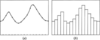Figure 1 (a) One-dimensional electron density and (b) its discrete version.

Define the function φ(x), shown in Fig. 2, by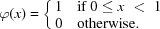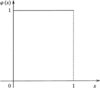Figure 2 Graph of the function, φ(x).

Then, since φ(xk) is only non-zero for kx < k + 1, we can write ρ(x) as a linear combination of these functions, i.e. we have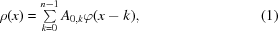where n is the number of grid points and A0,k is just the value of ρ at the kth grid point. If we now scale the function φ(x) by a factor of 2, then we can only obtain the approximation to the electron density given by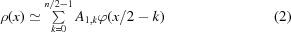as shown in the left-hand side of Fig. 3.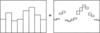Figure 3 The smoothed' electron density and the differences between this and the original density.

However, the differences between the approximation in (2) and the exact representation in (1) can be expressed in terms of the function ψ(x), shown in Fig. 4, defined by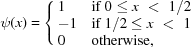so that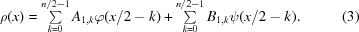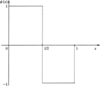Figure 4 Graph of the function, ψ(x).

Thus, if we add the two parts of Fig. 3, we again have an exact representation of our electron density (up to the sampling precision). We can now repeat the process on the smoothed' density in Fig. 3to obtain an even smoother version in terms of the functions φ(x/4 – k). Now, if we add two different levels of details we can again recreate our original density exactly, i.e. we have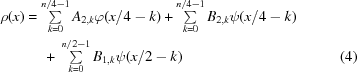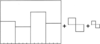Figure 5 The density after two levels of smoothing which would require two different scales of detail (as indicated) to be added in order to reconstruct the original density.

The function ψ(x) is the simplest wavelet function, known as the Haar wavelet. The function φ(x) is known as the Haar scaling function as it satisfies the equation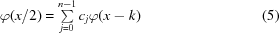(with c0 = c1 = 1), which tells us the relationship between the functions on one scale and those which are twice as wide. This may seem too obvious to express in this way, but the scaling equation in (5)holds for more complicated wavelet systems. In all cases, we represent our density as a linear combination of some wavelet-scaling function φ and its integer translates as in (1). The scaling equation (5)then allows approximations of the original density by functions scaled by a factor of 2. At each level, the differences between the approximations are stored in terms of wavelet functions ψ, related to φ through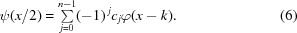In the usual Fourier representation, we express the electron density in terms of sines and cosines and each one contributes to every point in the map. In a wavelet representation, the functions φ and ψ are compactly supported; that is, each function is non-zero for a finite number of grid points. For example, the Haar scaling function is non-zero for a single grid point at the original scale. The number of coefficients cj in (5)is twice the number of non-zero grid points at the original scale. (The equation changes the scale by a factor of 2.) A set of wavelet functions is completely characterized by these coefficients, called filter coefficients to distinguish them from the wavelet coefficients Ai,k and Bi,k above. In fact, certain families of wavelet functions devised by Daubechies (1992) are referred to by the number of these coefficients. Daubechies' wavelets cannot be expressed as analytical formulae but are instead formed by an iterative procedure via (5). Daubechies' six-coefficient wavelet function, used in the phase-extension method, is shown in Fig. 6.Figure 6 A single wavelet function from the Daubechies' family. This six-coefficient wavelet function is the one currently used in the phase-extension method.

We have described the multi-resolution approach to wavelet analysis of Mallat (1989). In this case, the wavelet functions are specically constructed to give an exact representation at any level of the transform in terms of the functions φ and ψ associated with that level. Wavelet-type functions have also been considered in the crystallographic context by Lunin (2000) to provide an approximation to the electron-density distribution requiring few parameters.

### 3. Histogram matching

Histogram matching is a standard technique in image processing and has been used with success in X-ray crystallography. A histogram of density values can be calculated from any discrete image and compared with the histogram expected from a good' image. In the ab initio determination of molecular envelopes, this is used as a selection criteria in choosing phase sets from a large population of random starting phase sets (Lunin & Skovoroda, 1991). Furthermore, an image may be improved by systematically changing the density values to match the expected histogram; electron-density histogram matching plays an important role in density modification at high resolution (Zhang & Main, 1990). The technique depends on the availability of expected histograms and it has been shown that these can be predicted for unknown protein structures. At high resolution, the protein and solvent regions are easily identified and the histogram matching is only performed over the protein region. In this case the histograms depend only on resolution (Zhang, 1993). At very low resolution, the electron density from the entire unit cell must be used and molecular packing as well as the percentage of solvent play a part in determining the histogram. However, it has been found that only considering the asymmetric unit reduces the dependence on packing arrangements sufficiently and only resolution and solvent content need to be addressed (Main, 1998).

Mathematical models can be used to describe the histograms and Main (1990) provided a formula for calculating electron-density histograms at high resolution. Similarly, Lunin & Skovoroda (1991) suggested a two-component histogram model, calculated empirically to correspond with the histograms of known protein structures. At low resolution, the electron density from the entire cell must be included in the histogram, giving a bimodal distribution as shown in Fig.  7(a). Fig. 7(b) shows that the histograms can be described as a sum of two Gaussian functions, one which corresponds roughly to the solvent and one to the protein region.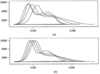Figure 7 Electron-density histograms at 9, 8, 7 and 6 Å (a) calculated from a typical protein after smoothing with a Gaussian kernel and (b) calculated from a two-Gaussian model.

The different levels of detail provided by the wavelet transform provides additional constraints on the electron density and allows histogram matching to be exploited further. For each of the xy and z directions, two levels of a wavelet transform are performed, giving sets of wavelet coefficients A2,k, B2,k and B1,k as in (4). The A2,k are the coefficients of the scaling functions and relate to the smooth version of the electron density. It is not surprising then that the histograms calculated from these coefficients look like low-resolution electron-density histograms. The histograms calculated from the detail coefficients B2,k and B1,k, however, look very similar to detail histograms obtained by Mallat (1989) for two-dimensional images. Mallat showed that these histograms can be described by the mathematical model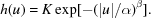The parameters α and β can be determined from the first and second moments of the histogram (see Wilson & Main, 2000). Fig. 8shows typical examples of detail histograms together with histograms calculated from Mallat's model; the histogram labelled D1 is calculated from the coefficients B1,k and the histogram labelled D2 from the coefficients B2,k. As the parameters depend only on resolution and percentage of solvent, we have been able to predict values for α and β as functions of resolution for different solvent contents. These histograms have been shown to work as efficiently as histograms calculated using the actual values of the coefficients.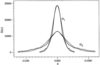Figure 8 Detail histograms from a two-level transform; D1 is calculated from the coefficients corresponding to the smallest details and D2 from the next level of detail. The thick lines show the actual histograms calculated from a typical protein after smoothing with a Gaussian kernel and the thin lines show the histograms predicted from Mallat's mathematical model.

### 4. Extending low-resolution phases

To form a starting point for the method, we have assumed that phases to 10 Å are available; an electron-density map is calculated with these. A two-level wavelet transform is then performed along the x direction to obtain sets of wavelet coefficients A2,k, B2,k and B1,k. The detail coefficients are then matched to values predicted for a slightly higher resolution, but the coefficients of the scaling functions A2,k are left unchanged. These coefficients provide the smoothed electron density to which the details are added and it has been found that not matching these coefficients allows them to keep their position and stabilizes the procedure. The inverse wavelet transform is then performed using the new coefficients and the electron density obtained is matched to the histogram predicted for it at the new resolution. As the wavelet transform is applied to the entire cell, the symmetry is destroyed and this is now reimposed and the difference between symmetry-related pixels is used to check the correctness of the map. The process is repeated until this difference is within pre-set limits. Wavelet transforms are also performed along the y and z directions (on the electron-density map from the start of the cycle) in the same manner to obtain three maps at the new resolution to be averaged. The Fourier magnitudes calculated from this averaged map are then compared with the observed magnitudes and an R factor is computed. The calculated magnitudes are replaced by the observed magnitudes and a new map calculated on which the whole process is repeated until the R factor shows convergence. This completes a single cycle of the phase extension as shown in the flowchart of Fig. 9. At this point, the resolution is increased and the procedure is repeated on the electron density calculated using the original 10 Å phases together with the new phases obtained in the previous cycle.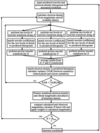Figure 9 Flowchart showing the various stages in the phase-extension procedure.

The two-Gaussian model used to describe the electron-density histograms allows systematic changes and improves the results. Decreasing the variance of the solvent distribution effectively leads to solvent flattening without the need to predesignate an area as solvent. Similarly, it has been found that sharpening the electron density in the protein region can be achieved by increasing the variance associated with the protein distribution.

### 5. Results

The method has been shown to work on a large number of model structures varying in size, solvent content and space group. Table 1shows the cumulative phase errors for a variety of proteins selected from the Protein Data Bank (Berman et al., 2000) for which structure factors were calculated from the atomic coordinates. There is a gradual build-up of phase errors as the calculation proceeds, but when calculated phases to 10 Å resolution are used we are currently able to extend these to a resolution of around 6–7 Å. In most cases, the 10 Å electron-density map is little more than a mask roughly covering the molecule (Fig. 10a), whereas in a 6 Å map it is often possible to identify secondary structure. Fig. 10(b) shows the 6 Å map after phase extension from 10 Å, where the mean error on the new phases is 73°. This can be compared with Fig. 10(c) which shows the 6 Å map calculated from the atomic coordinates.

 Table 1Cumulative mean phase errors (MPE) for new phases
 The resolution levels shown are representative and do not indicate the increments used.
PDB code Solvent (%) Symmetry MPE on phases (°) Starting phases New phases
10–9.0 Å 10–8.0 Å 10–7.0 Å 10–6.0 Å
1an8 75 P43212 60.2 60.6 68.8 72.4 356 1062
1cbf 69 P3121 48.9 58.0 68.7 77.8 273 901
1myg 65 I21 46.7 57.5 66.6 73.8 608 2019
1wsy 65 C2 54.0 65.0 73.0 79.5 890 3079
3gly 55 P212121 52.4 65.9 71.4 73.9 406 1211
2aai 54 P212121 48.9 60.3 70.1 76.8 440 1394
1olb 52 P212121 58.9 63.4 73.8 76.8 412 1271
1aac 51 P212121 54.5 63.7 70.9 76.4 504 1619
1ajg 50 P3121 53.5 66.6 72.8 78.2 311 1069
1alu 49 P3121 62.4 62.9 70.0 72.2 134 410
1an9 47 P212121 48.5 64.8 72.9 77.8 477 1546
1ako 47 P3121 57.9 58.2 70.3 79.8 187 598
1aqb 46 P212121 48.9 62.7 68.3 77.4 135 409
1al3 45 P21212 66.6 69.8 69.2 75.6 179 537
1am7 43 P212121 65.1 64.2 72.2 77.2 326 1024
†Number of independent starting reflections at 10 Å.
‡Number of independent new reflections, 10–6 Å.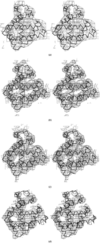Figure 10 Stereoviews showing electron-density maps for myoglobin (PDB code 1myg) with the Cα trace shown in thick lines. (a) 10 Å starting map calculated from the final model. (b) 6 Å map with phases between 10 and 6 Å given by our method. (c) 6 Å map calculated from the final model. (d) 6 Å map calculated after phase extension from starting phases with 46° mean error. All electron-density maps are calculated at 1.25σ, where σ is the r.m.s. deviation from the mean density of the map.

The method has also been used with experimentally measured magnitudes and we have found that the method works equally well if the data are complete. Fig. 11compares the results from calculated and experimental magnitudes and it can be seen that missing low-resolution data causes major problems. If, however, the experimental magnitudes are used where available and the missing data are replaced by appropriately scaled calculated magnitudes, the results are comparable to those obtained using only calculated magnitudes. All the graphs in Fig. 11show the results after phase extension where the 10 Å starting phases were calculated from the atomic coordinates. However, the method has also been shown to work when the starting phases have errors. A polyalanine model was created from an α-subunit of human haemoglobin and positioned in the myoglobin unit cell using the molecular-replacement program AMoRe (Navaza, 1990). The 10 Å starting phases calculated from this model have a mean phase error of 46° with respect to those calculated from the atomic coordinates of myoglobin. Again, the experimental magnitudes were used where available and calculated magnitudes were used for the missing reflections. Fig. 12shows the mean phase errors for the new phases in comparison with those obtained from perfect starting phases (i.e. those calculated from the final model).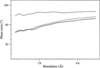Figure 11 Cumulative mean phase errors for myoglobin. The phase errors are shown when calculated magnitudes are used (thick line), when experimentally measured magnitudes are used where available and missing data are replaced with scaled calculated magnitudes (thin line) and when only the experimentally measured magnitudes are used (medium line). In this case ∼11% of the data below 10 Å were missing and the effect is dramatic. The phase errors given are for all new phases between 10 Å and the resolution shown.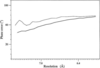Figure 12 Cumulative phase errors for myoglobin when starting phases have a mean phase error of 46° (thin line) in comparison to those when correct starting phases are used (thick line). In both cases the experimental magnitudes were used where available and missing data were replaced by scaled calculated magnitudes.

### 6. Phase refinement

As well as constraining the density by histogram matching and wavelet analysis, we are experimenting with applying constraints to the structure-factor phases. Some constraints are more easily applied in real space than reciprocal space and vice versa. We intend to exploit both. The assumption that the structure consists of randomly positioned atoms leads to the phase probability distribution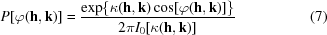where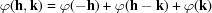and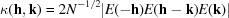with N equal atoms in the unit cell. This formula is the basis of the highly successful direct methods of crystal structure determination, which are successful at least when N is not too large and atomic resolution data are available.

For most proteins at less than atomic resolution, the formula gives little useful information. However, if more is known about the structure, it is possible to obtain new phase probability formulae that could be useful. For example, if the molecular envelope is known, the atoms can be assumed to be randomly distributed within the envelope instead of over the complete cell. This leads to the phase probability formula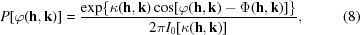where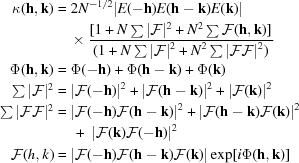with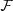(h) { = |(h)|exp[iΦ(h)]} being the Fourier coefficients of the volume containing the random atom distribution normalized to(0) = 1. Note that (8)indicates a most probable value for φ(h, k) of Φ(h, k), which is given by the phases of the appropriates. A combination of phase distributions gives a modified tangent formula which can express one phase in terms of a large number of others,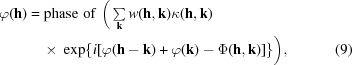where the weight w(h, k) takes account of the expected errors in the phases φ(h − k) and φ(k). With a small enough volume for the random atoms, this formula can give useful phase information for proteins, even at low resolution. Preliminary tests have achieved a phase improvement at 7 Å resolution from 62 to 53° for 480 strong reflections, using only a knowledge of the molecular envelope at 10 Å resolution.

If part of the secondary structure has been recognized from previous maps or a model for part of the structure is available, this can be included in a phase probability formula very similar to (8), but with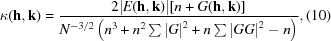where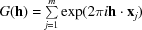and the same notation as given for (7) is used. There are m atoms in known positions, n randomly distributed (unknown) atoms and a total of N atoms in the cell. All atoms are assumed to be equal.

### 7. Discussion

In order to test the method when the starting phases have errors, we have used models which were suitable for molecular replacement; for example, the α-subunit of haemoglobin was used to provide phases for myoglobin. Although the phase error is as high as 46°, the models used are of sufficient quality for structure solution by the normal molecular-replacement method. However, since we only require phases to 10 Å, the method could be used in cases where the model is much poorer and the usual procedure fails. In particular, starting phases may be obtained from electron-microscopy images and work is in progress on an as yet unsolved protein for which 10 Å phases have been obtained by molecular replacement of such an image.

By 6–7 Å resolution, the build-up of phase errors is becoming too great and further information needs to be added to extend the phases further. At this stage, it is often possible to recognize secondary structure in the maps and it is hoped that this information can be recycled and the power of the method extended. Furthermore, the method should be improved significantly if an effective weighting scheme can be found, as none is used at present. The formulae given in the last section are currently being tested, but no results are yet available for publication. In addition, a formula has been developed which includes both known atomic positions and a restricted volume for the unknown atoms. It is intended that phase refinement using the above formulae will become part of the overall phase-determination procedure described in this paper.

### Acknowledgements

Julie Wilson is grateful to the BBSRC for the support afforded by the research grant 87/SBD7592.

### ReferencesBIOLOGICALCRYSTALLOGRAPHY